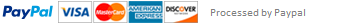### Mean and Median C program

Design a program that will allow some number of grades (up to a max of 100) to be input by the user. After the data has been collected, your program should calculate and output the mean and median of the collected data, as well as the sorted grade information.

Requirements

1. Use an integer constant of 100 to specify the number of elements in the array you will use to collect the grade information.

2. Declare any arrays you need in your main function.

3. Your data collection loop must allow the user to enter less than 100 grades. It must also make sure that the user does not try to enter more than 100 grades.

4. Each data value entered should be checked to make sure it is between 0 and 100 inclusive. Any other value entered should be considered invalid and ignored (ie. not counted as a valid input and not stored in the array).

5. Once the data is collected, the grades collected must be processed to find the mean. To find the mean, loop through the values in the array, summing them together. The mean is the sum divided by the number of grades. Output the mean in an appropriate manner (two digits after the decimal point).

6. Find the median of the grades. The median of a set of numbers is the number in the set where half the numbers are above it and half the numbers are below it. In order to find the median, the data in the array must be sorted.

7. The simplest sorting procedure is called bubble sorting. The following pseudocode describes bubble sorting for X array elements.

for outer = 0; outer < X; outer++

for inner = 0; inner < X-1; inner++

if array[inner] > array[inner+1]

// swap the array elements

tmp = array[inner]

array[inner] = array[inner+1]

array[inner+1] = tmp

8. After the data has been sorted, the median value can be found. If the array has an odd number of elements the median is the value of the middle element (Hint: number of grades / 2 is the middle element). If the array has an even number of elements then the median is the average of the middle two elements (Hint: number of grades / 2 and (number of grades / 2) – 1 are the two middle elements). The median value should be output in an appropriate manner.

9. Then main should output the sorted array with 5 grades per line in fields of 4 characters.

10. Carefully develop test cases for your program. Most of your test cases do not need to contain lots of values. Make sure to include incorrect inputs such as negative grade values. Calculate what your mean and median values should be for your test cases. Run your test cases with your program to see if your program generates the expected output. If not, troubleshoot your program and fix the problem.

11. Make sure that you code is properly formatted! Include comments that explain what your program is doing at each important step

This assignment has been answered 4 times in private sessions.